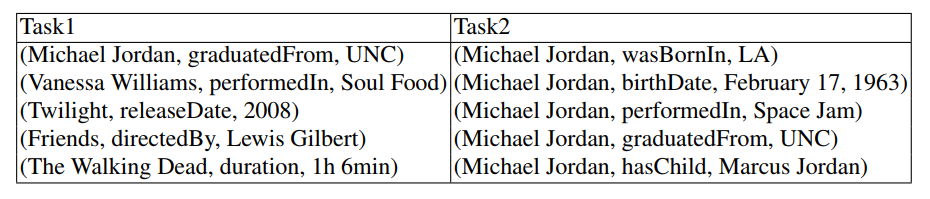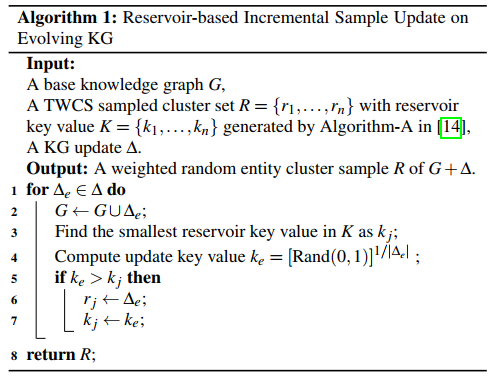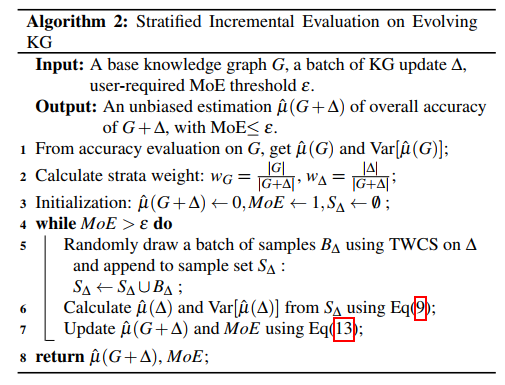# Efficient Knowledge Graph Accuracy Evaluation 论文笔记

## 抽样检测的思路

1. Simple Random Sampling

2. Cluster Sampling

motivation：标注员在标注三元组的时候，如果元组来自同一个实体，效率会高。理由是，只需了解一个对象，就可以标注多个三元组。

3. Weighted Cluster Sampling

4. Two-Stage Weighted Cluster Sampling

Motivation：随机抽取的实体，存在一些实体有着很多的三元组，如果这些元组全部都要判断的话，时间消耗非常多

5. Stratified Two-Stage Weighted Cluster Sampling

Motivation：实体的三元组所含有的数量越多，实体的正确率越高，方差越低。

## evolving KG 抽样检测的思路

evolving KG 表示正在不断更新的知识图谱，我们知道了原知识图谱的正确率，现在加入了新的三元组，我们需要确定加入新三元组之后，知识图谱的正确率。

1. 加权蓄水池算法(WRS)

WRS 中将更新 $\Delta$ 中的每一个实体视为一个新实体，不管这个实体是否已经存在于原来的知识图谱中。权重为一个实体的三元组个数，而不是占比。

2. 分层增量评估

## 置信区间

$E[\hat{\mu}] = \mu$

$\hat{\mu} = \frac{1}{n} \sum^{n}_{k=1} X_k \sim N(\mu, \frac{\sigma^2}{n})$

$\frac{\frac{1}{n} \sum^{n}_{k=1} X_k - \mu }{\frac{\sigma}{\sqrt{n}}} \sim N(0, 1)$

$P\{ -\frac{\bar{X} - \mu}{\frac{\sigma}{\sqrt{n}}} < z_\frac{\alpha}{2} < \frac{\bar{X} - \mu}{\frac{\sigma}{\sqrt{n}}} \} = 1 - \alpha$

$P\{ \bar{X}-\frac{\sigma}{\sqrt{n}}z_\frac{\alpha}{2} < \mu < \bar{X}+\frac{\sigma}{\sqrt{n}}z_\frac{\alpha}{2} \} = 1 - \alpha$

$\bar{X} \pm \frac{\sigma}{\sqrt{n}}z_\frac{\alpha}{2}$

## 时间消耗模型

1. 确定实体。这个实体指的是谁，比如下表中 Task 1 的 MJ 是科学家，而 Task 2 中的 MJ 是运动员。
2. 确定表述。确定三元组表述的事实是否正确。1. 不同实体越多，我们辨识实体消耗的时间越多。
2. 在总数不变的情况下，不同实体越少，实体的三元组越多，总的消耗时间越少，因为我们需要了解一个实体，就可以确定实体相关的三元组。

$cost(G') = |E'|\cdot c_1 + |G'| \cdot c_2$

$|E'|$表示不同实体个数，$|G'|$表示抽样产生的知识图谱个数。

$c_1$为确定实体消耗，$c_2$为确定表述消耗。在后面的实验中，得出了如下数据，$c_1 = 45, c_2 = 25$

1. 最优化的目标
2. 模拟实验中，可以拿来估计评估一组三元组需要的时间。我们可以通过合成三元组，再用这个模型计算消耗的时间，实验的时候人力都不需要了。

## 最优化问题

$min |E'|\cdot c_1 + |G'| \cdot c_2$

$st. E[\hat{\mu}] = \mu, MoE < \epsilon$

## Simple Random Sampling

$n_s$个随机变量为：

$t_1, t_2, ..., t_{n_s}$

$\hat{\mu_s} = \frac{1}{n_s} \sum^{n_s}_{i=1} f(t_i)$

$\hat{\mu_s} \pm z_{\frac{\alpha}{2}} \sqrt{\frac{\hat{\mu_s}(1-\hat{\mu_s})}{n_s}}$

$E[\hat{\mu_s}] = E[\frac{1}{n_s} \sum^{n_s}_{i=1} f(t_i)] = \frac{1}{n_s} \sum^{n_s}_{i=1} E[f(t_i)] = \frac{1}{n_s} \sum^{n_s}_{i=1} \mu = \mu$

cost 分析：

$n_c$ 表示不同实体的个数，$n_s$表示抽取三元组的总数

$cost = n_c \cdot c_1 + n_s \cdot c_2$

$n_c = X_1 + X_2 + ... + X_N$

$E[n_c] = E[\sum^{N}_{i = 1} X_i] = \sum^{N}_{i=1} E[X_i] = \sum^{N}_{i=1} P\{抽n_s次抽到第 i 个实体\} = \sum^{N}_{i=1} (1 - (1 - \frac{M_i}{M})^{n_s})$

$cost = \sum^{N}_{i=1} (1 - (1 - \frac{M_i}{M})^{n_s}) \cdot c_1 + n_s \cdot c_2$

$\frac{\sigma}{\sqrt{n_s}}z_\frac{\alpha}{2} \le \epsilon$

$n_s \ge \frac{\hat{\mu_s}(1-\hat{\mu_s})z^2_{\alpha_2}}{\epsilon^2}$

cost 随着 $n_s$ 增大而增大，所以取下界，即是理论上，最少的采样数量。

$n_s = \frac{\hat{\mu_s}(1-\hat{\mu_s})z^2_{\alpha_2}}{\epsilon^2}$

## Random Cluster Sampling

n 个随机变量，$\tau_{I_k}$表示第$I_k$个实体的正确三元组个数：

$\frac{N}{M}\tau_{I_1}, \frac{N}{M}\tau_{I_2}, ..., \frac{N}{M}\tau_{I_n}$

$\hat{\mu_r} = \frac{N}{Mn} \sum^{n}_{k=1} \tau_{I_k}$

$\hat{\mu_s} \pm z_{\frac{\alpha}{2}} \sqrt{\frac{1}{n(n-1)}\sum^n_{k=1}(\frac{N}{M}\tau_{I_k}-\hat{\mu_r})^2}$

$E[\tau_{I_k}] = \frac{1}{N} (M_1 * \mu_1 + M_2 *\mu_2 + ... + M_N * \mu_N) = \frac{1}{N} * \mu * M$

$E[\hat{\mu_r}] = E[\frac{N}{Mn} \sum^{n}_{i=1} \tau_{I_k}] = \frac{N}{Mn} \sum^{n}_{i=1} E[\tau_{I_k}] = \frac{N}{Mn} \sum^{n}_{i=1} \frac{M}{N} * \mu = \mu$

## Weighted Cluster Sampling

n 个随机变量，$\mu_{I_k}$ 表示第 $I_k$ 个实体的正确率：

$\mu_{I_1}, \mu_{I_2}, ..., \mu_{I_n}$

$\hat{\mu_w} = \frac{1}{n} \sum^{n}_{k=1} \mu_{I_k}$

$\hat{\mu_w} \pm z_{\frac{\alpha}{2}} \sqrt{\frac{1}{n(n-1)}\sum^n_{k=1}(\mu_{I_k}-\hat{\mu_r})^2}$

$E[\mu_{I_k}] = \sum^{N}_{i=1} \frac{M_i*\mu_i}{M} = \mu$

$E[\hat{\mu_w}] = E[\frac{1}{n} \sum^{n}_{k=1} \mu_{I_k}] = \frac{1}{n} \sum^{n}_{k=1} E[\mu_{I_k}] = \mu$

## Two-Stage Weighted Cluster Sampling

n 个随机变量，每个随机变量为随机抽样 m 个得到的正确率：

$\hat\mu_{I_1}, \hat\mu_{I_2}, ..., \hat\mu_{I_n}$

$\hat\mu_{w,m} = \frac{1}{n} \sum^{n}_{k=1} \hat\mu_{I_k}$

$\hat\mu_{w,m} \pm z_{\frac{\alpha}{2}} \sqrt{\frac{1}{n(n-1)}\sum^n_{k=1}(\hat\mu_{I_k}-\hat\mu_{w,m})^2}$

$E[\hat\mu_{w, m}] = \frac{1}{n} \sum^{n}_{k=1} E[\hat\mu_{I_k}] = \frac{1}{n} \sum^{n}_{k=1} \sum^{N}_{i=1} \frac{M_i}{M} \mu_i = \frac{1}{n} \sum^{n}_{k=1} \mu = \mu$

cost 分析：

$\hat\mu_{w, m} \sim N(\mu, Var(\hat\mu_{w, m}))$

$\hat\mu_{w, m} \pm z_{\frac{\alpha}{2}} \sqrt{Var(\hat\mu_{w, m})}$

$z_{\frac{\alpha}{2}} \sqrt{Var(\hat\mu_{w, m})} \le \epsilon$

$n \ge \frac{V(m)z_{\frac{\alpha}{2}}^2}{\epsilon^2}$

## Stratified Two-Stage Weighted Cluster Sampling

$\hat\mu_{ss} = \sum_h W_h \hat\mu_{w,m,h}$

## Reservoir Incremental Evaluation

WRS 中的加权，权重是一个实体的三元组个数 $M_i$。一开始一直在纠结用比例 $M_i / M$，一直没搞懂如何才能保持比例不变呢。 WRS 中将 $\Delta$ 中的每一个实体视为一个新实体，不管这个实体是否已经存在于原来的知识图谱中。

WRS 的结果为按权重抽样的结果，计算正确率的时候使用 WCS 的公式即可。当然，某个更新可能包含一个实体的几百个三元组，此时可以对这个样本进行简单随机抽样，计算正确率，再用 TWCS 的公式来计算。## Stratified Incremental Evaluation## 实验中重要的结论

cost function

Oracle Stratification

static KG evaluation

TWCS 对比 SRS，TWCS 抽取的实体种类少，TWCS 相比 SRS 大概节约 20% 的时间。但是，在高准确率的情况下，TWCS 又是并不明显，有的甚至比 SRS 慢。

TWCS 中 m 的数值

in practice, cluster size may serve as a good signal indicating similarities among entity accuracies for large clusters but not for those small ones, and the overall variance is not reduced as we expected.

TWCS 评估时间的影响因素

TWCS 评估的时间不受知识图谱的大小影响！！！

TWCS 评估的时间受到知识图谱正确率的影响，在 50% 正确率的情况下，达到最大。

Single Batch of Update

RS 能够 fault-tolerant。实验结果表明，如果一开始知识图谱的正确率评估是不准确的，那么再接下来的更新中，RS 能够较快的进入实际的正确率，但是 SS 一直受到之前结果的影响，很难达到实际的正确率。

## Q&A

1，每个抽样方法的 cost 分析得出的抽样数量究竟有什么用？比如，SRS 中我们抽样了 $n_s$ 个，得到了均值，再用这个均值反推 $n_s$，这不就死锁了吗？再比如，TWCS 中我们需要知道知识图谱每个实体的准确率，整体的正确率，我们才能求 $n, m$，我们去优化这个问题干什么呢？

2，RCS 和 WCS 的 cost 如何分析？

3，为什么 TWCS 需要计算出方差，而不用样本方差呢？

$cost = n \cdot c_1 + \sum^n_{k=1} min\{M_{I_k}, m\} \cdot c_2$

## 参考文献

posted @ 2020-08-02 17:38  楷哥  阅读(158)  评论(4编辑  收藏